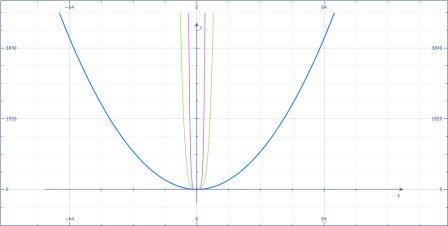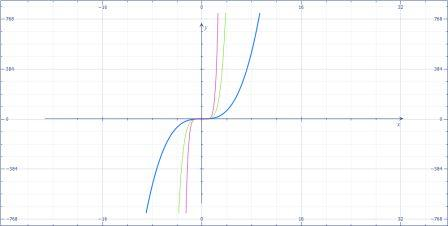# Consider the function f(x)=x^n for positive integer values of n. \\ (a) For what values of n does...

## Question:

Consider the function {eq}f(x)=x^n {/eq} for positive integer values of {eq}n {/eq}.

(a) For what values of {eq}n {/eq} does the function have a relative minimum at the origin?

(b) For what values of {eq}n {/eq} does the function have a point of inflection at the origin?

## Concavity and Relative Extremes

This problem is purely based on the understanding of the behavior of functions. While solving this problem we can consider some examples, we can draw some graphs to justify our answers. The solution to this problem is purely based on reasoning.

The function that we have is,

{eq}\displaystyle f(x)=x^n {/eq}

(a) For what values of {eq}n {/eq} does the function have a relative minimum at the origin?

So, we need a minimum at the origin. If our function turns out to be a curve that has a minimum at the origin we will be able to answer. Such functions could be,

{eq}\displaystyle f(x)=x^2 {/eq} or {eq}\displaystyle f(x)=x^4 {/eq} or {eq}\displaystyle f(x)=x^6 {/eq}

The graph below shows the minimum of such functions at the origin.• {eq}\displaystyle \boxed{\displaystyle \text{So, we can see that for all the positive even integer values of n the minimum lies at the origin }} {/eq} OR {eq}\displaystyle \text{positive even integer values of } n {/eq}.

(b) For what values of {eq}n {/eq} does the function have a point of inflection at the origin?

Now, we need a point of inflection at the origin. We know that,

• {eq}\displaystyle \text{Point of inflection is a point where the concavity of the function changes or the direction of slope of the tangent changes}. {/eq}

So, we need a sign change in the direction of slope at the origin. Let us have a look on the set of graphs of the functions {eq}\displaystyle f(x)=x^3, f(x)=x^5,f(x)=x^7 {/eq}below:In the graph we can see that for the odd values of {eq}n>1 {/eq} we have functions whose point of inflection lies at the origin. We have considered {eq}n>1 {/eq} because if {eq}n=1 {/eq} it will be a straight line.

• {eq}\displaystyle \boxed{\displaystyle \text{ So for the set of positive odd integers }\text{the point of inflection lies at the origin}} {/eq} OR {eq}\displaystyle \text{ the values of n should be odd positive integers}. {/eq}